Debug Numerical Issues in Fixed-Point Conversion Using Variable Logging

This example shows some best practices for debugging your fixed-point code when you need more than out of the box conversion.

Convert to Fixed Point Using Default Configuration

1. From the apps gallery, open the Fixed-Point Converter app.

2. On the Select page, browse to the kalman_filter.m file and click .

3. Click Next. On the Define Input Types page, browse to the kalman_filter_tb file. Click .

The test file runs and plots the input noisy signal, the filtered signal, the ideal filtered signal, and the difference between the filtered and the ideal filtered signal.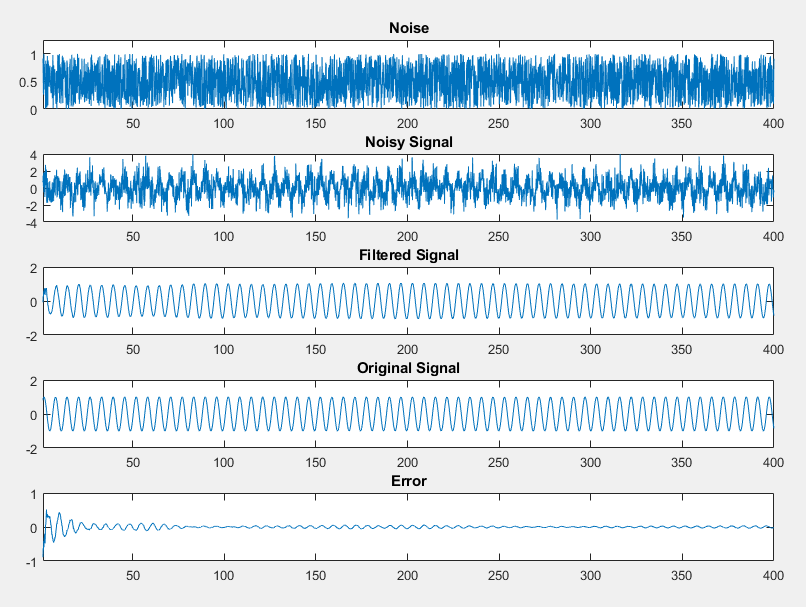4. Click . On the Convert to Fixed Point page, click Analyze to gather range information and data type proposals using the default settings.

5. Click Convert to apply the proposed data types.

6. Click the Test arrow and select the Log inputs and outputs for comparison plots check box. Click Test. The Fixed-Point Converter runs the test file kalman_filter_tb.m to test the generated fixed-point code. Floating-point and fixed-point simulations are run, with errors calculated for the input and output variables.

The generated plots show that the current fixed-point implementation does not produce good results.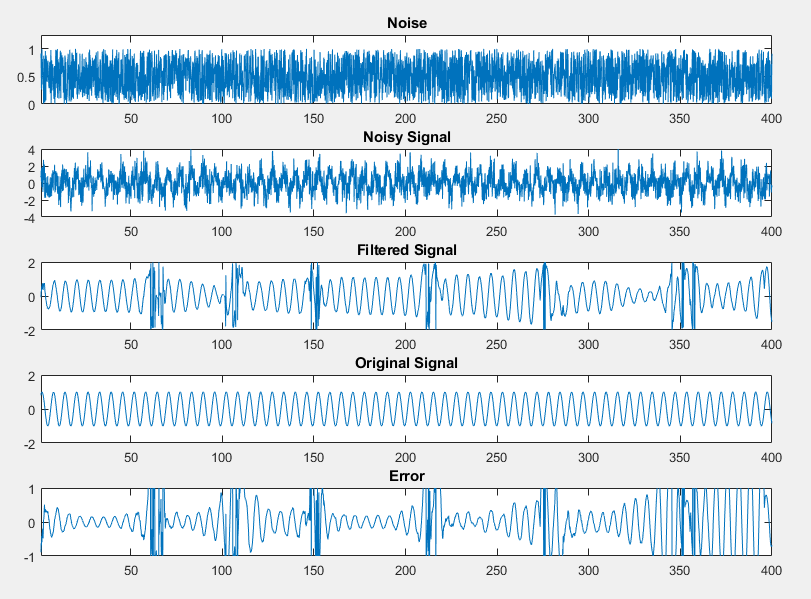The error for the output variable y is extremely high, at over 282 percent.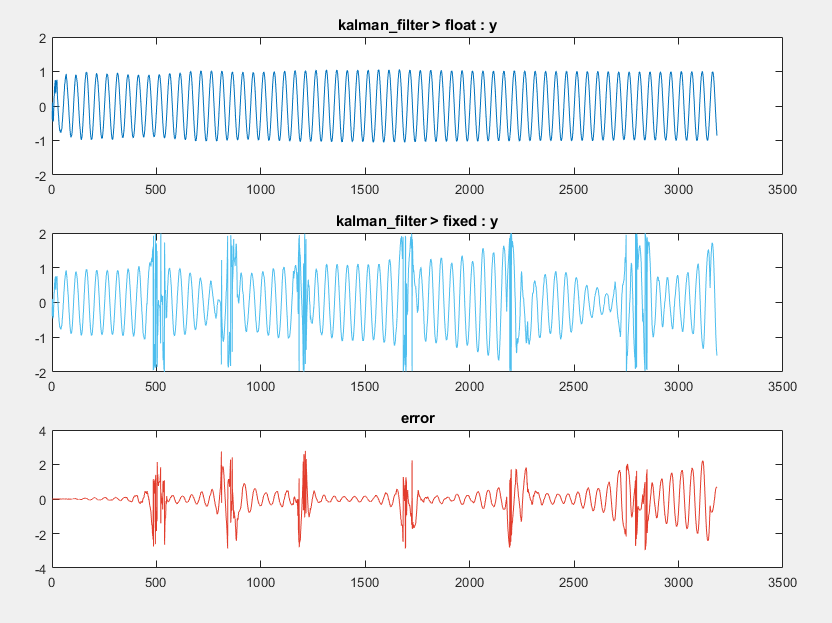Determine Where Numerical Issues Originated

Log any function input and output variables that you suspect are the cause of numerical issues to the output arguments of the top-level function.

1. Click kalman_filter in the Source Code pane to return to the floating-point code.

When you select the Log inputs and outputs for comparison plots option during the Test phase, the input and output variables of the top level-function, kalman_filter are automatically logged for plotting.

2. The kalman_filter function calls the matrix_solve function, which contains calls to several other functions. To investigate whether numerical issues are originating in the matrix_solve function, select kalman_filter > matrix_solve in the Source Code pane.

In the Log Data column, select the function input and output variables that you want to log. In this example, select all three, a, b, and x.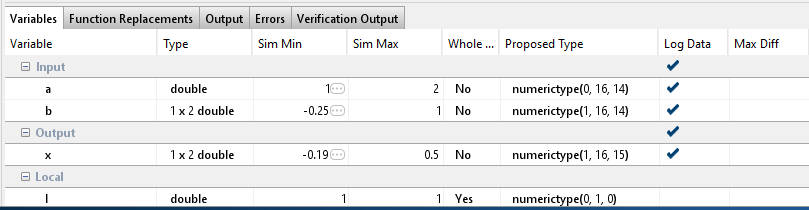3. Click .

The generated plot shows a large error for the output variable of the matrix_solve function.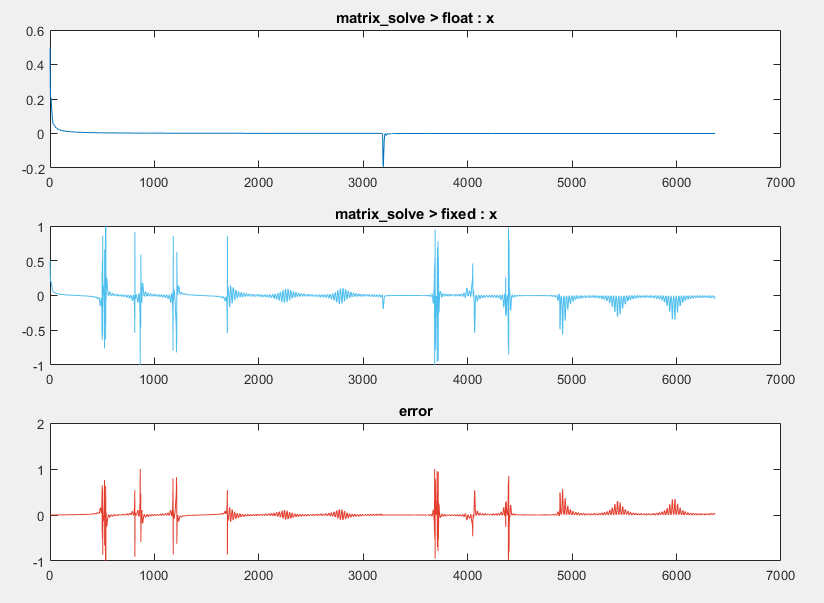1. On the Convert to Fixed Point page, click Settings.

Under fimath, set the Rounding method to Nearest. Set the Overflow action to Saturate.

2. Click to apply the new settings.

3. Click the arrow next to and ensure that Log inputs and outputs for comparison plots is selected. Enable logging for any function input or output variables. Click .

Examine the plot for top-level function output variable, y.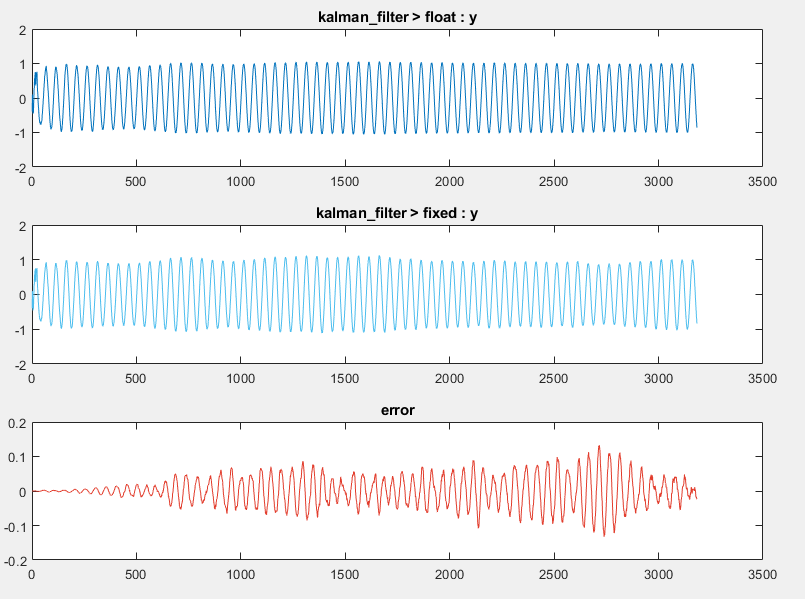The new fimath settings improve the output, but some error still remains.

Adjusting the default word length improves the accuracy of the generated fixed-point design.

1. Click and change the default word length to 32. Click to apply the new settings.

2. Click . The error for the output variable y is accumulating.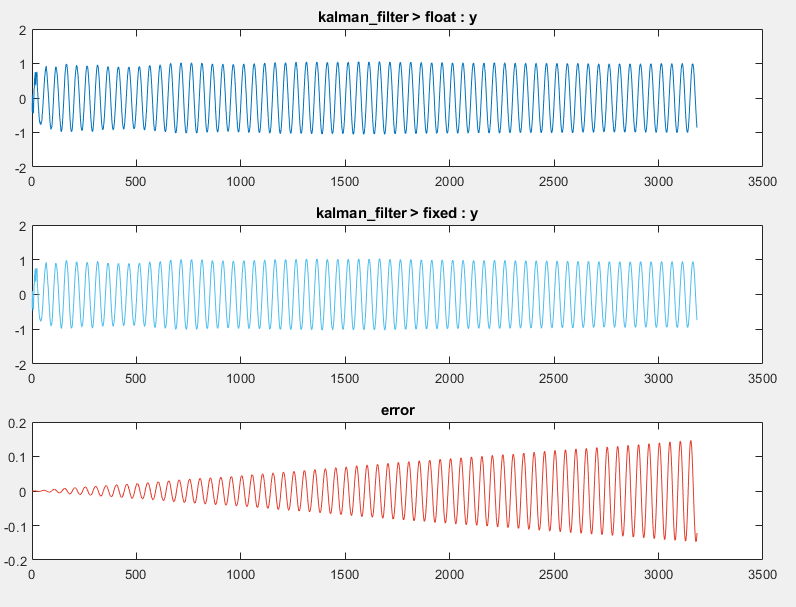3. Close the Fixed-Point Converter and plot window.

Replace Constant Functions

The kalman_stm function computes the state transition matrix, which is a constant. You do not need to convert this function to fixed point. To avoid unnecessary quantization through computation, replace this function with a double-precision constant. By replacing the function with a constant, the state transition matrix undergoes quantization only once.

1. Click the kalman_filter function in the Source Code pane. Edit the kalman_filter function. Replace the call to the kalman_stm function with the equivalent double constant.

A = [0.992114701314478, -0.125333233564304; 0.125333233564304, 0.992114701314478];
Save the changes.

2. Click Analyze to refresh the proposals.

3. Click Convert to apply the new proposals.

4. Click Test. The error on the plot for the functions output y is now on the order of 10-6, which is acceptable for this design.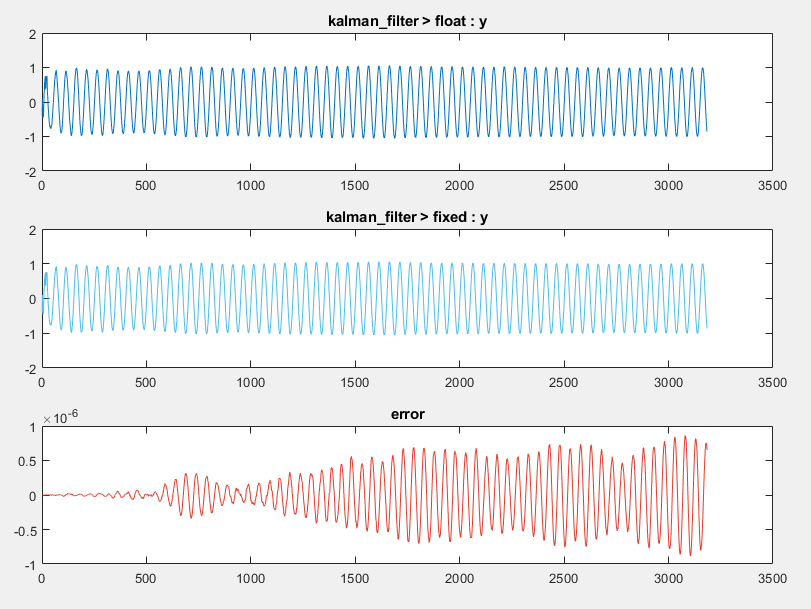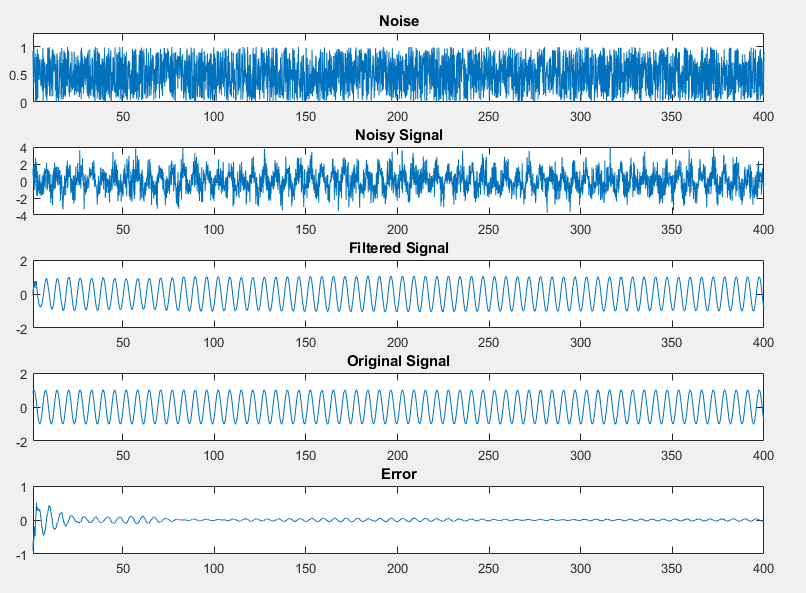Fixed-Point Designer DocumentationGet trial now